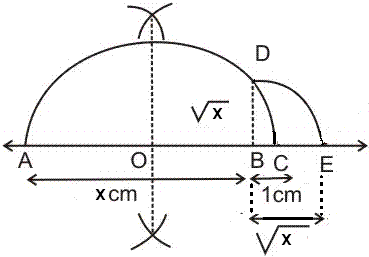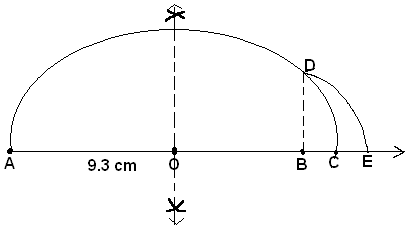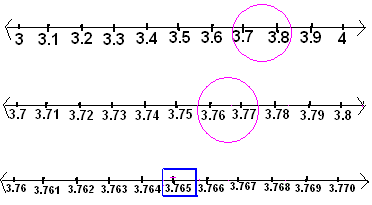Courses

# Representation of Real Numbers on number line & Operations on real numbers Notes | EduRev

## Class 9 : Representation of Real Numbers on number line & Operations on real numbers Notes | EduRev

The document Representation of Real Numbers on number line & Operations on real numbers Notes | EduRev is a part of the Class 9 Course Mathematics (Maths) Class 9.
All you need of Class 9 at this link: Class 9

For the representation of real numbers on the number line, use the following steps:
Represent √x on the number line.

Step 1: Draw a line and mark a point A on it.
Step 2: Mark a point B on the line drawn such that AB = x cm.
Step 3: Mark a point C on AB produced such that BC = 1 cm.
Step 4: Find mid-point of AC (x+1). Let the mid-point be O.
Step 5: Taking O as centre and OC = OA [(x+1)/2] as radius draw a semi-circle. Also draw a line passing through B perpendicular to OB. Let it cut the semi-circle at D
Step 6: Taking B as the centre and BD as radius draw an arc cutting OC produced at E.Point E so obtained represents √x.Examples:

Represent √(9.3) on the number line.

Step 1: Draw a line and mark a point A on it.
Step 2: Mark a point B on the line drawn such that AB = 9.3cm.
Step 3: Mark a point C on AB produced such that BC = 1 cm.
Step 4: Find mid-point of AC (9.3+1). Let the mid-point be O.
Step 5: Taking O as center and OC = OA [10.3/2] as radius draw a semi-circle. Also draw a line passing through B perpendicular to OB. Let it cut the semi-circle at D
Step 6: Taking B as the center and BD as radius draw an arc cutting OC produced at E.Point E so obtained represents √(9.3).Visualize 3.765 on the number line using successive magnification.

Step 1: Since the given number lies between 3 and 4, look at the portion of the number line between 3 and 4.
Step 2: Divide the portion between 3 and 4 into 10 equal parts and mark each point of the division as shown in Fig. 1.
Step 3: The 7th mark and 8th mark of this sub-division corresponds to 3.7 and 3.8 respectively and 3.765 lies between them (3.7 < 3.765 < 3.8)
Step 4: Again divide the portion between 3.7 and 3.8 into 10 equal parts. Now 3.765 lies between its 6th and 7th mark (3.76 < 3.765 < 3.77).
Step 5: Divide the portion between 3.76 and 3.77 again into ten equal parts. Therefore, the 5th mark of this sub-division mark represents 3.765 as shown in fig.3Offer running on EduRev: Apply code STAYHOME200 to get INR 200 off on our premium plan EduRev Infinity!

## Mathematics (Maths) Class 9

46 videos|323 docs|108 tests

,

,

,

,

,

,

,

,

,

,

,

,

,

,

,

,

,

,

,

,

,

;